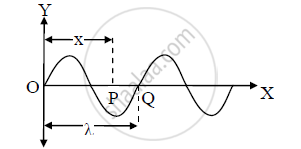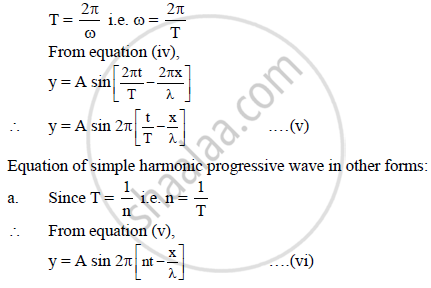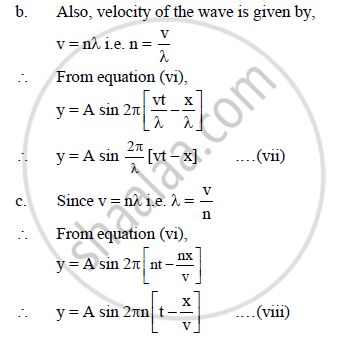# Derive an Expression for One Dimensional Simple Harmonic Progressive Wave Travelling in the Direction of Positive X-axis. Express It in ‘Two’ Different Forms. - Physics

Derive an expression for one dimensional simple harmonic progressive wave travelling in the
direction of positive X-axis. Express it in ‘two’ different forms.

#### Solution

Equation of simple harmonic progressive wave:a) Consider a simple harmonic progressive wave travelling in the direction of the positive X-axis. The vibrations of the particles of the medium is parallel to the Yaxis.

b) At time t = 0, particle is at origin O i.e., the mean position. At instant ‘t’,
displacement of the particle is,

y = A sin ωt ….(i)

where, A = amplitude,

ω = angular velocity

c) Consider a particle P situated at a distance ‘x’ from O. If ‘δ’ be the phase difference between the particle P and O, then displacement of the particle of the medium at P in instant ‘t’ is given by,

y = A sin (ωt − δ) .…(ii)

d) A path difference of λ between two particles of a medium corresponds to a phase difference of 2π between them (e.g. particle O and Q). Since the path difference between P and O is ‘x’, so the phase difference ‘δ’ between them is given by,

δ = (2pix)/lambda   ....(iii)

Substituting equation (iii) in equation (ii) we have

y = A sin[ωt - (2pix)/lambda]  ....iv

This equation gives the displacement of any particle of the medium at any instant. Hence, it is called equation of a simple harmonic progressive wave.

e) SinceAll the above equations represent one-dimensional simple harmonic progressive wave,
travelling in the direction of the positive X-axis.

Concept: Simple Harmonic Progressive Waves,
Is there an error in this question or solution?
2012-2013 (October)

Share